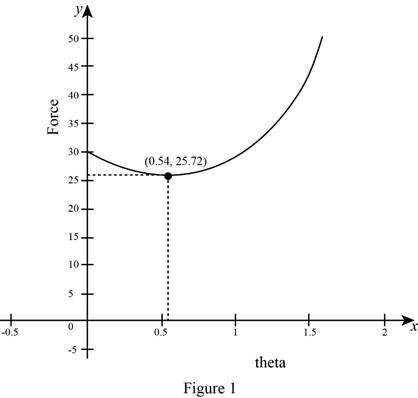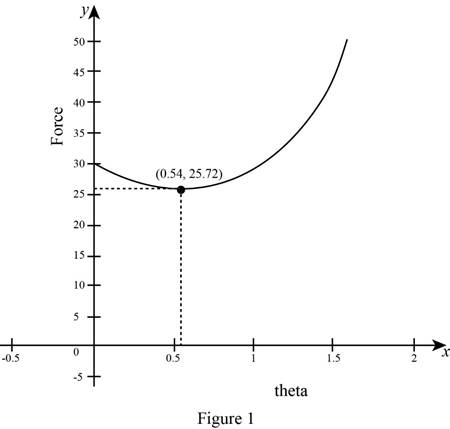# The rate of change of F with respect to θ . That is, d F d θ .### Single Variable Calculus: Concepts...

4th Edition
James Stewart
Publisher: Cengage Learning
ISBN: 9781337687805### Single Variable Calculus: Concepts...

4th Edition
James Stewart
Publisher: Cengage Learning
ISBN: 9781337687805

#### Solutions

Chapter 3.3, Problem 38E

(a)

To determine

## To find: The rate of change of F with respect to θ. That is,dFdθ.

Expert Solution

The rate of change of F with respect to θ is dFdθ=μW(sinθμcosθ)(μsinθ+cosθ)2_.

### Explanation of Solution

Given:

The magnitute of the force is F=μWμsinθ+cosθ,

Where, μ is a costant and W is weight.

Derivative Rule: Quotient Rule

If f(θ). and g(θ) are both differentiable function, then

ddθ[f(θ)g(θ)]=g(θ)ddθ[f(θ)]f(θ)ddθ[g(θ)][g(θ)]2 (1)

Calculation:

Obtain the derivative of F.

dFdθ=ddθ(F)=ddθ(μWμsinθ+cosθ)

Apply Quotient Rule as shown equation (1),

dFdθ=(μsinθ+cosθ)ddθ[μW]μWddθ[μsinθ+cosθ][μsinθ+cosθ]2=(μsinθ+cosθ)μW[μcosθsinθ][μsinθ+cosθ]2=0μW[μcosθsinθ][μsinθ+cosθ]2=μW[sinθμcosθ][μsinθ+cosθ]2

Therefore, the rate of change of F with respect to θ is dFdθ=μW[sinθμcosθ][μsinθ+cosθ]2_.

(b)

To determine

### To find: The value of θ if the rate of change equal to zero.

Expert Solution

The rate of change equal to zero when θ=arctanμ_.

### Explanation of Solution

Given:

From part (a), the rate of change of F with respect to θ is dFdθ=μW[sinθμcosθ][μsinθ+cosθ]2.

Calculation:

The rate of change equal to zero, dFdθ=0.

μW[sinθμcosθ][μsinθ+cosθ]2=0μW[sinθμcosθ]=0

Since μW0, sinθμcosθ=0.

sinθ=μcosθsinθcosθ=μtanθ=μθ=arctan(μ)

Therefore, the rate of change equal to zero when θ=arctanμ_.

(c)

To determine

Expert Solution

### Explanation of Solution

Given:

The magnitute of the force is F=μWμsinθ+cosθ, W=50 lb and μ=0.6.

Use online graphing calculator to draw the graph of F as a function of θ as shown in Figure 1.The value of θ is appoximately 0.54.

Calculation:

Obtain the magnitute of the force F as a function of θ.

F=μWμsinθ+cosθ (2)

Substitute W=50 lb and μ=0.6 in equation (2),

F=(0.6)(50)(0.6)sinθ+cosθ=30(0.6)sinθ+cosθ

Therefore, the magnitute of the force F as a function of θ is F=30(0.6)sinθ+cosθ.

From part (b), the rate of change equal to zero when θ=arctanμ.

Substitute μ=0.6 in equation θ=arctanμ,

θ=arctan0.60.54

Therefore, the value of θ is appoximately 0.54.

Substitute θ0.54 in s and obtain the value of F.

F=30(0.6)sin(0.54)+cos(0.54)=30(0.6)(0.51414)+(0.85771)=301.16619425.72

Therefore, the point (θ,F)(0.54,25.72)

Use online graphing calculator and draw the graph of F as a function of θ as shown below in Figure 1.From Figure 1, it is observed that the slope of tangent is horizontanl to the curve F=30(0.6)sinθ+cosθ at the point (0.54,25.72).

Hence, the value of θ is consistent.

### Have a homework question?

Subscribe to bartleby learn! Ask subject matter experts 30 homework questions each month. Plus, you’ll have access to millions of step-by-step textbook answers!